# Using the Analysis Toolpak Data Analysis Tools

The Analysis Toolpak add-in is part of the deal when you purchase Excel 2007, although you might not know it. Add-ins are little packages of tools that more or less seamlessly integrate into the user interface of Excel. However, they require you to install them first. The following sections discuss the installation of, and the tools included with, the Analysis Toolpak.

### Installing the Analysis Toolpak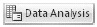To see whether you have the Analysis Toolpak installed, click the Data tab on the Ribbon. If the Data Analysis button is there, then you're good to go. If not, click the Microsoft Office Button, click Excel Options, and select the Add-Ins category. In the Manage list at the bottom of the dialog box, select Excel Add-Ins, and then click the Go button to display the dialog box shown in Figure 17-6. In the Add-Ins dialog box, select the Analysis Toolpak check box, and then click OK to install it. Excel prompts you for permission to proceed. Click Yes.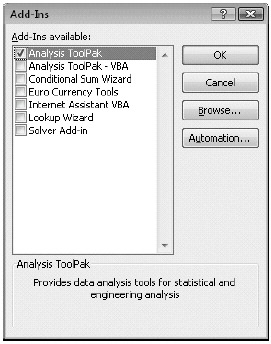Figure 17-6: Use the Add-Ins dialog box to install the Analysis Toolpak.

When you click the Data Analysis button on the Data tab, the Data Analysis dialog box appears, as shown in Figure 17-7.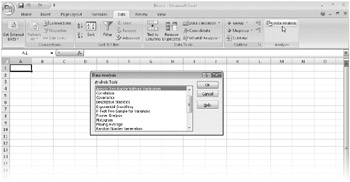Figure 17-7: Click the Data Analysis button on the Data tab to display the Data Analysis dialog box.

### Using the Descriptive Statistics Tool

The Descriptive Statistics tool provides a table of statistics for one or more sets of input values, showing the general tendencies and variability of the data. For each variable in the input range, this tool's output range includes a detailed list of statistics, as shown in Figure 17-8. To use the Descriptive Statistics tool, click the Data Analysis button on the Data tab on the Ribbon, select Descriptive Statistics, and click OK. The Descriptive Statistics dialog box shown in Figure 17-8 appears.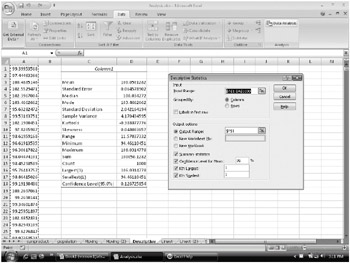Figure 17-8: We generated this table of statistics describing the column of 1,000 values contained in Column A using the Descriptive Statistics tool.

The Descriptive Statistics tool requires an input range that consists of one or more variables and an output range. You must also indicate whether the variables are to be arranged by column or by row. If you include a row of labels, be sure to select the Labels In First Row option. Excel then uses the labels to identify the variables in its output table. Select the Summary Statistics option only if you want a detailed output table as shown in Figure 17-8; otherwise, leave this check box empty.

Like the other tools in the Analysis Toolpak, Descriptive Statistics creates a table of constants. If a table of constants doesn't suit your needs, you can obtain most of the same statistical data from other Analysis Toolpak tools or from formulas that use the Excel worksheet functions. Table 17-2 lists the statistics and formulas.

Table 17-2: Descriptive Statistics FormulasOpen table as spreadsheet

Statistic

Formula

Mean

=AVERAGE(number1, number2, . . .)

Standard error

Similar to =STEYX (known_y's, known_x's) but uses the ±-distribution rather than the standard regular distribution.

Median

=MEDIAN(number1, number2, . . .)

Mode

=MODE(number1, number2, . . .)

Standard deviation

=STDEV(number1, number2, . . .)

Variance

=VAR(number1, number2, . . .)

Kurtosis

=KURT(number1, number2, . . .)

Skewness

=SKEW(number1, number2, . . .)

Range

=MAX(number1, number2)-MIN (number1, number2, . . .)

Minimum

=MIN(number1, number2, . . .)

Maximum

=MAX(number1, number2, . . .)

Sum

=SUM(number1, number2, . . .)

Count

=COUNT(value1, value2, . . .)

kth largest

=LARGE(array, k)

kth smallest

=SMALL(array, k)

Confidence

Similar to =CONFIDENCE (alpha, standard_dev, size) but uses a different algorithm

### Creating Histograms

A histogram is a chart (usually a simple column chart) that takes a collection of measurements and plots the number of measurements (called the frequency) that fall within each of several intervals (called bins).

To see how the Histogram tool works, we'll use a table of 1,000 test scores. (The input range must contain numeric data only.) To see a breakdown of the total scores at 50-point intervals, begin by setting up the distribution bins shown in column F of Figure 17-9.Figure 17-9: Column F contains the distribution bins.

The distribution bins don't have to be equally spaced like the ones in Figure 17-9 are, but they must be in ascending order. Click the Data Analysis button on the Data tab, select the Histogram tool, and then click OK. Figure 17-10 shows the Histogram dialog box.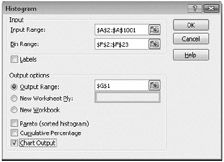Figure 17-10: This dialog box appears after you select the Histogram tool in the Data Analysis dialog box.

The Histogram tool can take three items of information: the location of the data (in this case, D2:D1001), the location of the bins (F2:F22), and the upper-left cell of the range where you want the analysis to appear (G1). After you click OK, Excel writes its analysis in columns G and H, as shown in Figure 17-11.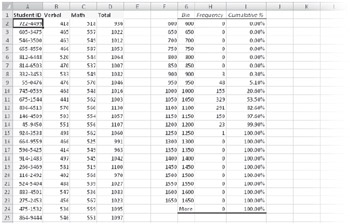Figure 17-11: This analysis tells us that three scores were at least 900 but less than 950; 48 are at least 950 but less than 1,000; and so on.

 Note We created our own distribution bins for this model, but you can let the Histogram tool determine the divisions for you. Leave the Bin Range box blank to create evenly distributed bin intervals using the minimum and maximum values in the input range as beginning and ending points. The number of intervals is equal to the square root of the number of input values.

Here are a few facts to keep in mind when using the Histogram tool:

• In the Frequency column, the histogram reports the number of input values that are equal to or greater than the bin value but less than the next bin value.

• The last value in the table reports the number of input values equal to or greater than the last bin value.

• Select the Pareto check box in the Histogram dialog box to sort the output in descending order.

• Select the Cumulative Percentage option to create a table that lists the cumulative percentages of each bin level.

• If you select the Chart Output option in the Histogram dialog box, the Histogram tool simultaneously generates a chart and places it next to the frequency distribution table.

For everything you need to know about charts, see Part 6, "Creating Charts."

Inside Out-Beware of Bin FormulasNotice that the Histogram tool duplicates your column of bin values in the Bin column, which is convenient if you place the output somewhere else in your workbook. But because the Histogram tool copies the bin values, it's best if the bin range contains numeric constants rather than formulas. If you do use formulas, be sure they don't include relative references; otherwise, when Histogram copies the range, the formulas might produce unwanted results.#### Analyzing Distribution with the FREQUENCY Function

It's easy to generate a new frequency distribution table using the Histogram tool whenever you change the input values, but the Histogram tool generates static numbers (numeric constants). If you'd rather create formulas linked to the input values, you can use the built-in FREQUENCY array function, which calculates the number of times specified values occur in a population and takes the arguments (data_array, bins_array) Figure 17-12 shows the FREQUENCY function applied to the data shown in Figure 17-9.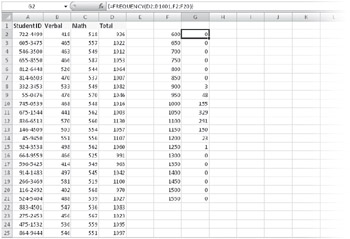Figure 17-12: Use the FREQUENCY function to link the distribution analysis to the input data.

To use the FREQUENCY function, set up a column of bin values, just as you would with the Histogram tool, and then select the entire range where you want the output to appear, which in our example would be G2:G21-the cells in column G that are directly adjacent to the bin values in column F. (This range must be a column, because FREQUENCY can't use a row or multicolumn range as its output range.) Then type the formula, specifying the input range as the first argument and the bin range as the second. Press Ctrl+Shift+Enter to lock in the array formula. For more information about arrays, see "Using Arrays" on page 468.

### Using the Rank And Percentile Tool

Suppose you want to rank the scores shown in Figure 17-9. You could rank them by sorting the data in descending order, with the best score at the top and the worst score at the bottom of the column. To find the rank of any score, you might want to create an ascending series of numbers beside the sorted scores, with 1 beside the best score and 1,000 beside the worst.

The Rank And Percentile tool not only performs these tasks for you but also creates percentile figures for each value in your input range. To use this tool, click the Data Analysis button on the Data tab on the Ribbon, select Rank And Percentile, and then click OK. Figure 17-13 shows the Rank And Percentile dialog box.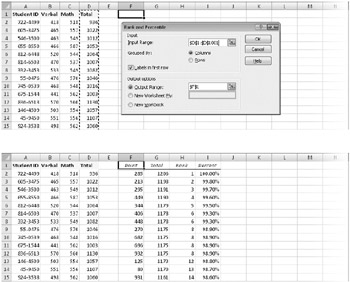Figure 17-13: Use the Rank And Percentile tool to generate an output table like the one shown in the lower figure.

 Note If the Data Analysis button does not appear on the Data tab, see "Installing the Analysis Toolpak"on page 567.

Here's how to read the output of the Rank And Percentile tool, shown at the bottom of Figure 17-13. The first row of the output table (F2:I2) tells us that the 285th item in the input range is a total score of 1,206, which ranks first and is better than 100 percent of the other scores. Here are some hints to remember when using the Rank And Percentile tool:

• It's best to use the Labels In First Row option in the Rank And Percentile dialog box and then include the column heading in the input range. This way, the second column in the output table uses the same label. If you do not include the label in the input range, the output column is labeled Column1.

 Note If you select the Labels In First Row option but do not actually include the cell containing the label in your input range, the first value in the input range becomes the title. For example, if the input range in Figure 17-13 were \$D\$2:\$D\$1001, the resulting label in column G would be 936 instead of Total.
• In Figure 17-13, we analyzed a single column of data, but we could analyze the Verbal, Math, and Total columns together. In that case, we would specify the input range B1:D1001, and the tool would generate 12 columns of output, lour lor each input column.

• You can also have the output table placed on a new worksheet or workbook, which is a good idea if you select multiple columns of input data resulting in a large output table.

### Generating Random Numbers

The built-in random-number function, RAND, generates a uniform distribution of random real numbers from 0 to 1. In other words, all values from 0 to 1 share the same probability of being returned by a set of formulas based on the RAND function. Because the sample is relatively small, the distribution is by no means perfectly uniform. Nevertheless, repeated tests demonstrate that the RAND function doesn't favor any position within its spectrum of distribution. For more information, see "The RAND and RANDBETWEEN Functions" on page 500.Correlating Tables

The input and output tables, shown at the bottom of Figure 17-13, share a common column of data-the Total column-and the same number of rows. But because the two tables are sorted differently, the rows don't match. The easiest solution is to sort the output table by the Point column; in this context, Point indicates the position of the corresponding data point in the input range. Therefore, sorting the output table by the Point column puts it in the same order as the input table: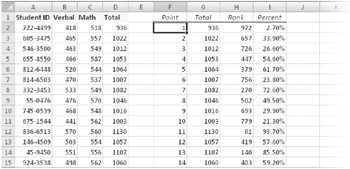If you want to add information from the output table to the existing input table, you can delete the Point column (because the Point column simply indicates the row number), the Total column (because the input table already has a Total column), and the blank column in the output table, creating a single, correlated table.TROUBLESHOOTING Random numbers keep changing. The RAND function is one of Excel's volatile functions-that is, it recalculates every time the worksheet recalculates, which happens every time you make an entry in a cell. If you want to generate a set of random numbers and then "freeze" them, select all the RAND formulas in your worksheet, and press Ctrl+C to copy them. Then click the Paste button on the Home tab on the Ribbon, and click Paste Values to replace the volatile formulas with fixed values. Or, instead of using the RAND function, use the Random Number Generation tool (described next), which produces constants instead of formulas.

The Random Number Generation tool creates sets of random numbers that are not uniformly distributed. You can then use the Histogram tool to sort and plot the results for Monte Carlo decision analysis and other kinds of simulations. Six distribution types are available: Uniform, Normal, Bernoulli, Binomial, Poisson, and Discrete (user-defined). In addition, you can select Patterned in the Distribution list to create nonrandom numbers at specified intervals. Click the Data Analysis button on the Data tab on the Ribbon, select Random Number Generation, and then click OK to display a dialog box like the one shown in Figure 17-14.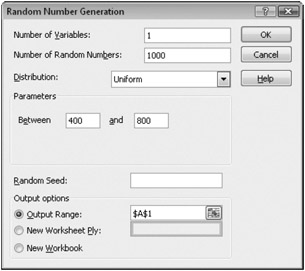Figure 17-14: The Parameters area in the Random Number Generation dialog box changes to reflect the distribution type you select.

Here are a couple of important points regarding using the Random Number Generation tool:

• In the Number Of Variables and Number Of Random Numbers text boxes, you indicate how many columns of numbers you want and how many numbers you want in each column. For example, if you want 10 columns of 100 numbers each, specify 10 in the Number Of Variables text box and 100 in the Number Of Random Numbers text box.

• You can also specify a seed value. However, each time you generate a random-number set with a particular distribution type using the same seed value, you get the same sequence of numbers; therefore, you should specify a seed value only if you need to be able to reproduce a random-number sequence.

#### Random Number Distribution Methods

In the Random Number Generation dialog box, the parameters shown directly below the Distribution list change, depending on the type of distribution you select. As Figure 17-14 shows, when you select Uniform in the Distribution list, you can specify the beginning and ending points of the distribution in the Between and And text boxes.

#### Distributing Random Numbers Uniformly

This option asks you to specify two numbers between (and including) which to generate a set of random numbers, and it works much the same way as the RANDBETWEEN function, generating an evenly distributed set of real numbers. You can use this option as a more convenient alternative to RAND if you want endpoints other than 0 and 1 or if you want sets of numbers to be based on the same seed value.

#### Distributing Random Numbers Normally

Normal distribution has the following characteristics:

• One particular value, the mean, is more likely to occur than any other value.

• Values greater than the mean are as likely to occur as values less than it.

• Values close to the mean are more likely to occur than values distant from the mean.

To generate normally distributed random numbers, you specify two parameters: the mean and the standard deviation. The standard deviation is the average absolute difference between the random numbers and the mean. (Approximately 68 percent of the values in a normal distribution will fall within one standard deviation of the mean.)

#### Generating Random Numbers Using Bernoulli Distribution

The Bernoulli Distribution option simulates the probability of success of a number of trials, given that all trials have an equal probability of succeeding and that the success of one trial has no impact on the success of subsequent trials. (Note that success in this context has no value implication. In other words, you can use this distribution to simulate failure as readily as success.) All values in the Bernoulli distribution's output are either 0 or 1.

The probability that each cell will return a 1 is given by the distribution's sole parameter-P Value-for which you supply a number from 0 to 1. For example, if you want a sequence of 100 random Bernoulli values whose most likely sum is 27, you define a 100-cell output range and specify a P Value of 0.27.

#### Generating Random Numbers Using Binomial Distribution

The Binomial Distribution option simulates the number of successes in a fixed number of trials, given a specified probability rate. As with the Bernoulli Distribution option, the trials are assumed to be independent; that is, the outcome of one has no effect on any other. To generate binomially distributed numbers, you specify Number Of Trials and the P Value (probability) that any trial will succeed. (Again, success in this context has no value implication. In other words, you can use this distribution to simulate failure as readily as success.)

For example, suppose you make 10 sales presentations a week, you close the sale 20 percent of the time, and you would like to know what your success rate might be over the next year. Type 50 (for 50 working weeks in the year) in the Number Of Random Numbers text box, 0.2 in the P Value text box, and 10 in the Number Of Trials text box to learn that you can expect to make no sales four weeks in the coming year.

#### Generating Random Numbers Using Poisson Distribution

The Poisson Distribution option simulates the number of times an event occurs within a particular time span, given a certain probability of occurrence. The occurrences are assumed to be independent; that is, each occurrence has no effect on the likelihood of others.

The Poisson Distribution option takes a single parameter, Lambda, which represents the expected outcome of an individual occurrence. For example, suppose you receive an average of 10 service calls a day. You want to know how often you can expect to get 18 or more service calls in a day over a year. To get this information, type 260 (52 weeks times 5 days) in the Number Of Random Numbers box and 10 in the Lambda box (the expected average). You can then use the COUNTIF function to count the number of times 18 shows up in the output range. For more information, see "The SUMIF, SUMIFS, and COUNTIF Functions" on page 555.

#### Generating Random Numbers Using Discrete Distribution

Use the Discrete Distribution option to create a custom distribution pattern by specifying a table of possible outcomes along with the probability associated with each outcome. The probability values must be from 0 to 1, and the sum of the probabilities in the table must equal 1. To use the Discrete Distribution option, specify the possible outcomes and their probabilities as a two-column range whose reference is the only parameter used by this option.

For example, you could create a custom distribution pattern to generate random snow-shovel sales patterns based on a two-column input range: Month Number and Probability of Snow.

#### Generating Semi-Random Numbers Using Patterned Distribution

Selecting Patterned in the Distribution list in the Random Number Generation dialog box generates numbers that are both random and part nonrandom. Selecting the Patterned option displays the dialog box shown in Figure 17-15.Figure 17-15: The Patterned option in the Distribution list creates an arithmetic series with operational repetitions.

You can think of the Patterned option as a fancy Fill Series command. It creates one or more arithmetic series with optional internal repetitions. For example, to create the series shown in Figure 17-15, complete the dialog box as shown, requesting two sequences of the numbers 1 through 10, using a step interval of 3, and repeating each number twice within each cycle.

For more information, see "Filling and Creating Data Series" on page 211.

If the step interval takes the series beyond the specified upper value, the output range includes the upper value because the last interval is truncated. For example, if you specify a step interval of 4 and the numbers 1 through 10, Excel creates the series 1, 5, 9, and 10.

### Sampling a Population of Numbers

The Sampling tool extracts a subset of numbers from a larger group (or population) of numbers. From an input range, you can sample a specified number of values at random or at every nth value. The Sampling tool copies the extracted numbers to an output range you specify. Click the Data Analysis button on the Data tab on the Ribbon, select Sampling, and then click OK to display the Sampling dialog box, like the one shown in Figure 17-16.Figure 17-16: The Sampling tool extracts a random or periodic subset of a numeric population.

The values in the input range must be numeric. They can include blank values and dates, provided you type the dates as numbers, not text. For example, to simplify a chart of daily commodity prices, you can use the Sampling tool to extract every nth data point and then create a new plot from the extracted data.

 Note To perform the equivalent of sampling from a range containing text values, set up a series of ascending integers beginning at 1 in a column alongside the text values, and then use the Sampling tool to extract numbers from this series. Then you can assemble a list of sampled text values by using the resulting numbers as arguments to the INDEX function. For more information, see "The INDEX Function" on page 517.

### Calculating Moving Averages

A moving average is a forecasting technique that simplifies trend analysis by smoothing fluctuations that occur in measurements taken over time. These fluctuations can be caused by random noise that is often a by-product of the measurement technique. For example, measurements of the height of a growing child will vary with the accuracy of the ruler and whether the child is standing straight or slouching. You can take a series of measurements, however, and smooth them over time, resulting in a curve that reflects the child's actual growth rate. Fluctuations in measurements can result from other temporary conditions that introduce bias. Monthly sales, for example, might vary with the number of working days in the month or the absence of a star salesperson who takes a vacation.

Suppose you have created the 18-month demand curve shown in Figure 17-17. To generate a less noisy trendline from this data, you can plot a six-month moving average. The first point in the moving average line is the average of the first six monthly figures (January through June 2008). The next point averages the second-through-seventh monthly figures (February through July 2008), and so on. You can use the Moving Average tool to perform this analysis for you. Click the Data Analysis button on the Data tab on the Ribbon, select Moving Average, and then click OK to display the Moving Average dialog box, as shown in Figure 17-18.Figure 17-17: We'll use this 18-month demand curve to demonstrate the Moving Average tool.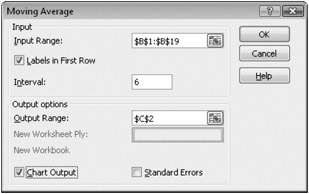Figure 17-18: The Moving Average tool helps smooth out bumpy curves to reveal the trend.

The Moving Average tool requires three pieces of information: the input range that contains the data you want to analyze, the output range where the averaged data will appear, and the interval over which the data is averaged. To determine a three-month moving average, for example, specify an interval of 3.

Figure 17-19 shows a six-month moving average superimposed over the original demand curve in Figure 17-18. The Moving Average tool produced the data in column C, which was used to create the straighter plot line in the chart. Notice that the first five cells in the tool's output range contain #N/A error values. Where the interval is n, you will always have n-1 #N/A error values at the beginning of the output. Including those values in a chart presents no problem, because Excel leaves the first area of the plot line blank.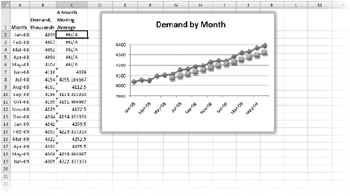Figure 17-19: The Moving Average tool provides a better perspective of the overall trend.

In Figure 17-19 we applied some judicious formatting to the rather plain chart generated by the Moving Average tool. To learn more about charts, see Part 6, "Creating Charts."

Notice that each cell containing a moving average value in Figure 17-19 displays a flag in the upper-left corner of the cell. This is an error flag; after you select the cell, a smart tag action menu appears alerting you that the formula omits adjacent cells. In this case, it's OK. To remove the flags, select all the flagged cells, click the smart tag to display its menu, and then choose the Ignore Error command.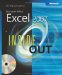MicrosoftВ® Office ExcelВ® 2007 Inside Out (Inside Out (Microsoft))
ISBN: 073562321X
EAN: 2147483647
Year: 2004
Pages: 260

Similar book on Amazon